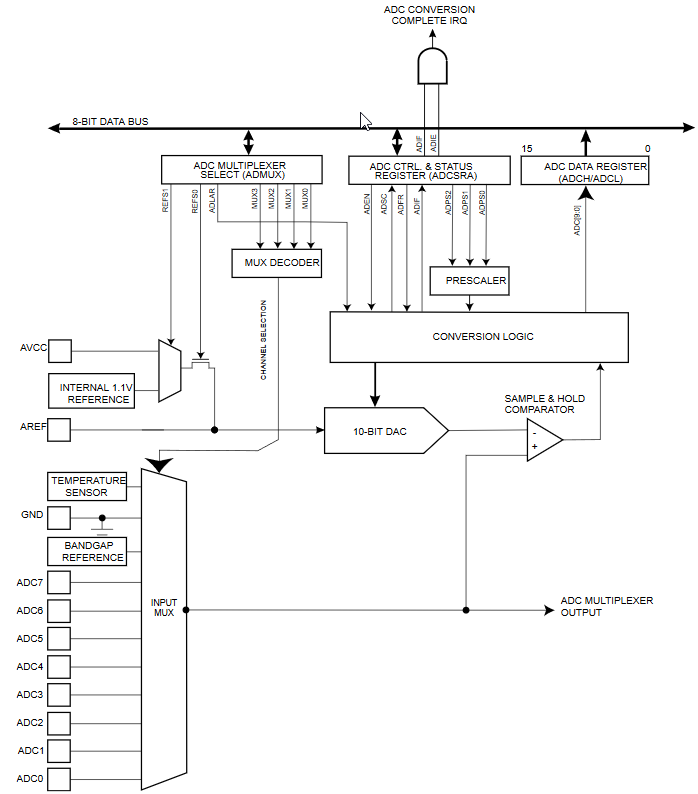# A/D Converter - Free Running Mode

Zápisník experimentátora

Hierarchy:

The A/D converter has one interesting option to measure the analog signal as quickly as possible. Starting a new analogue measurement can be triggered by the end of the previous measurement. This mode is called `Free Running`.## Example

The Free Running mode is set in the `ADCSRB` register. All ADTS[0-2] bits must be set to 0. The first measurement must be run by setting the bit `ADSC`. It is also necessary to set the bit `ADATE`, which invokes the automatic startup according to the set ADTS bit combination.

In order not to be an entirely boring example, using the variable `counter` in the interrupt, I count how many measurements Arduino did in one second. In such a clock setting, Arduino will make about 9600 measurements in one second. If you need more measurements then you can manipulate the prescaler with bits `ADPS`. Without more serious problems with reduced accuracy, you can set the value to 16 to get about 77,000 measurements per second.

``````const byte adc_pin = A0; // = 14 (pins_arduino.h)
volatile unsigned long counter = 0;
unsigned long counter_last = 0;

void setup() {
Serial.begin (115200);

| ((adc_pin - 14) & 0x07); // Arduino Uno to ADC pin
}

void loop() {
uint8_t SREG_old = SREG;
noInterrupts();
unsigned long cnt = counter;
SREG = SREG_old;

Serial.print(", counter = ");
Serial.print(cnt);
Serial.print(" (+");
Serial.print(cnt - counter_last);
Serial.println(")");
counter_last = cnt;
delay(1000);
}